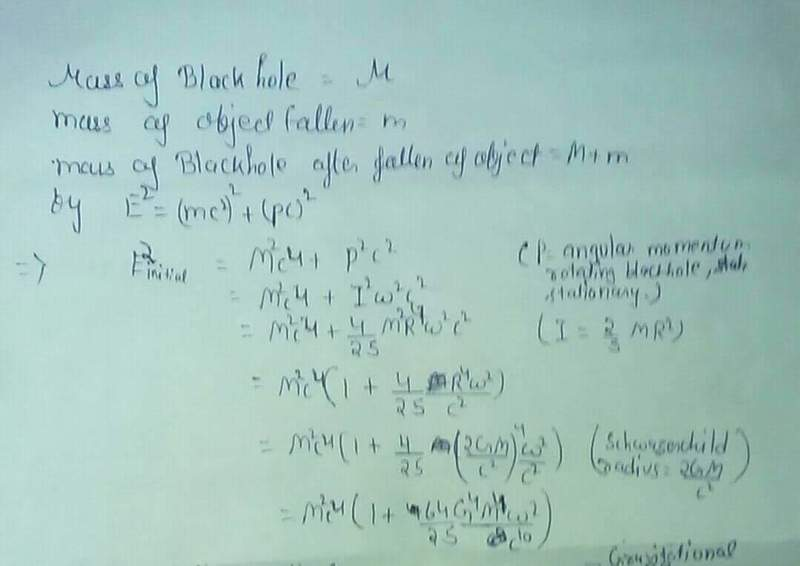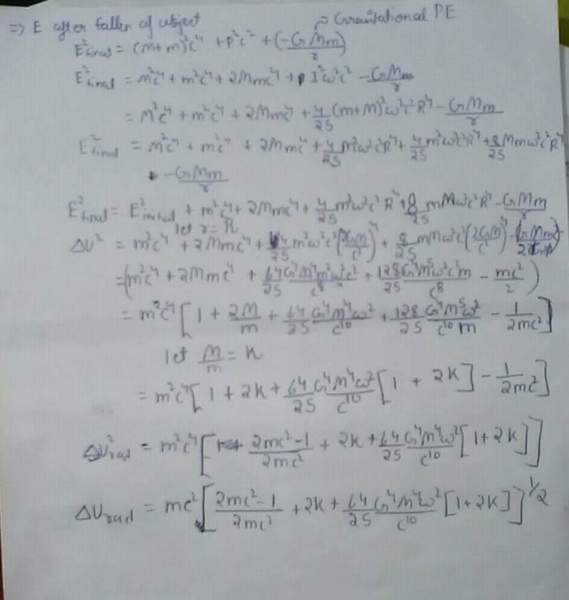# Energy Radiated by Object Falling in Black Hole

• I
• Riyan
In summary: In fact, they are so good that one common approximation is to use Post Newtonian methods for all non-relativistic objects, rather than resorting to more accurate methods only when dealing with black holes.In summary, Post Newtonian approximation is not applicable to a black hole, and will produce inaccurate results.

#### Riyan

I'm trying to find how much energy will radiate when an object fell in a black hole.
Is my calculations are correct or I'd committed mistake somewhere?#### Attachments

Last edited by a moderator:
Welcome to the PF.

You dropped a squared term on the 14th line.

@Riyan, according to PF rules, you should be posting your equations directly using the PF LaTeX feature, not as an image. Please see here for help on how to do that:

https://www.physicsforums.com/help/latexhelp/

Doing this will make it much easier to help you since we will be able to quote particular equations directly.

berkeman said:
Welcome to the PF.

You dropped a squared term on the 14th line.
Which term?

Riyan said:
Which term?
I was obviously joking. As Peter says, we expect math to be posted using LaTeX so it is clear and easy to follow and easy to quote. Please use the tutorial that he linked to in order to post a reply with all of your work in LaTeX. Learning LaTeX is a good thing -- it is a skill that you will use a lot in your technical career.At a conceptual level, I see a basic problem in your work. You are using special relativity formulas and Newtonian gravitational potential energy. These are both completely inapplicable to the situation. The simplest correct way to do this would be to use Post Newtonian approximation (linearized GR would not work because it doesn’t handle gravitational radiation).

This is not a simple undertaking. An example introduction to the field is:

https://www.physics.uoguelph.ca/poisson/research/postN.pdf

However, some simplification is possible in your scenario - the simplest body in GR is a BH, so handling the infall of a small body into a BH is equivalent to the so called extreme mass ratio BH merger. This allows so called effective one body methods, as discussed here:

https://arxiv.org/abs/gr-qc/0612096

Admittedly, this A level material not I level, but I don’t think there is meaningful way to handle the problem without advanced techniques.

Last edited:
PAllen said:
The simplest correct way to do this would be to use Post Newtonian approximation

This won't work for a black hole. It will for for an object falling onto, say, the Earth from a high altitude, but a black hole cannot be described in the Post Newtonian approximation; you would need to use the full Schwarzschild metric.

berkeman said:
I was obviously joking. As Peter says, we expect math to be posted using LaTeX so it is clear and easy to follow and easy to quote. Please use the tutorial that he linked to in order to post a reply with all of your work in LaTeX. Learning LaTeX is a g,ood thing -- it is a skill that you will use a lot in your technical career.I'll post it as soon as possible.

•berkeman
PeterDonis said:
This won't work for a black hole. It will for for an object falling onto, say, the Earth from a high altitude, but a black hole cannot be described in the Post Newtonian approximation; you would need to use the full Schwarzschild metric.
How would I use schwarzschild metric in this ?

Riyan said:
How would I use schwarzschild metric in this ?

It depends on what kind of scenario you have in mind. There are at least two fairly simple scenarios:

(1) An object on some free-fall trajectory in the gravitational field of the black hole. In this case, the Schwarzschild metric can be used to derive an effective potential similar to the one used in Newtonian mechanics. (In the simplest case of radial free fall, i.e., zero angular momentum, this effective potential is formally the same as the one in Newtonian mechanics, but the symbol ##r## does not stand for the radial distance, it is the radial coordinate of the Schwarzschild metric.)

(2) An object that is brought to rest at some finite altitude above the hole's horizon. In this case, the Schwarzschild metric gives a simple formula for the energy this object has, as measured at infinity, as compared with its rest energy (the energy it would have if it were at rest next to an observer at infinity). This formula is the same as the formula for gravitational time dilation/redshift.

Note that in case #1, if the object is allowed to freely fall radially into the hole, it does not radiate any energy at all. In case #2, the object will have to radiate energy, and the formula tells you how much.

PeterDonis said:
This won't work for a black hole. It will for for an object falling onto, say, the Earth from a high altitude, but a black hole cannot be described in the Post Newtonian approximation; you would need to use the full Schwarzschild metric.
You would think this is true from the derivation of Post Newtonian methods, but in fact they do work remarkably well for black hole mergers:

https://arxiv.org/abs/1102.5192

PeterDonis said:
It depends on what kind of scenario you have in mind. There are at least two fairly simple scenarios:

(1) An object on some free-fall trajectory in the gravitational field of the black hole. In this case, the Schwarzschild metric can be used to derive an effective potential similar to the one used in Newtonian mechanics. (In the simplest case of radial free fall, i.e., zero angular momentum, this effective potential is formally the same as the one in Newtonian mechanics, but the symbol ##r## does not stand for the radial distance, it is the radial coordinate of the Schwarzschild metric.)

...

Note that in case #1, if the object is allowed to freely fall radially into the hole, it does not radiate any energy at all. In case #2, the object will have to radiate energy, and the formula tells you how much.

This is also, perhaps surprisingly, not true:

https://arxiv.org/abs/1012.2028

PAllen said:
You would think this is true from the derivation of Post Newtonian methods, but in fact they do work remarkably well for black hole mergers

Hm, interesting. Another ugly belief spoiled by a beautiful theory.PAllen said:
This is also, perhaps surprisingly, not true

Yes, you're right, what I said is strictly true only for an actual test particle that has negligible mass, not just compared to the black hole mass, but in the sense that it produces no detectable spacetime curvature. The paper you link to is treating the more realistic case of an object with small mass compared to that of the hole, but still large enough to produce detectable curvature, which is treated as a perturbation on the Schwarzschild metric (but only to first order, so it doesn't change the particle's trajectory).

PeterDonis said:
what I said is strictly true only for an actual test particle that has negligible mass, not just compared to the black hole mass, but in the sense that it produces no detectable spacetime curvature

Btw, for purposes of the question posed by the OP, I would strongly recommend sticking to this idealized case rather than trying to treat the more realistic case discussed in the paper (for one thing, the latter can only be solved numerically).

So where am I wrong :
1. From the beginning
2. or, I took wrong gravitational PE

Riyan said:
So where am I wrong

Once more: you need to post your equations. Then we can quote them directly and help you. We can't quote anything from the image you posted.

Or, if posting the equations is too much, at the very least you can describe your solution strategy in words, step by step. Then we can at least quote the steps that might have issues.

Mass of black hole =M
Mass of object =m
Mass of object after fallen of object =M+m
\begin{align}
E^2 &=M^2 c^4+p^2 c^2
\\ &=M^2 c^4+I^2 \omega^2 c^2
\\&=M^2 c^4+\frac {4M^2 r^4 \omega^2 c^2 }{25}
\\&=M^2 c^4(1+\frac {4r^4 \omega^2} {25 c^2} )
\end{align}

Mass of black hole =M
Mass of object =m
Mass of object after fallen of object =M+m
Initial energy of black hole:
\begin{align}
E^2 &=M^2 c^4+p^2 c^2
\\ &=M^2 c^4+I^2 \omega^2 c^2
\\&=M^2 c^4+\frac {4M^2 r^4 \omega^2 c^2 }{25}
\\&=M^2 c^4(1+\frac {4r^4 \omega^2} {25 c^2} )
\end{align}
\begin{align}
=M^2 c^4(1+\frac {4 ( \frac {2 G M} {c^2} )^4 \omega^2} {25 c^2} )
\\&=M^2 c^4(1+ \frac {64 G^4 M^4 \omega^2} {25 c^{10}} )
\end{align}

Riyan said:
Mass of black hole =M
Mass of object =m
Mass of object after fallen of object =M+m

Right here you have assumed that there is zero radiation. If the object radiates any energy away while it is falling in, then it cannot add its full mass ##m## to the black hole; that would violate conservation of energy. So these equations say that the object freely falls into the hole and emits zero radiation, adding its full mass to the mass of the hole.

Riyan said:
Initial energy of black hole

Should just be ##Mc^2## (or ##M## if you use units where ##c = 1##, which is common, and highly recommended, in relativity problems). Spacetime is not flat, so you cannot use the equations of special relativity. The best you can do is to work in coordinates in which the black hole is at rest, and in those coordinates its energy is just its mass.

In your (2) and (6), what is ##\omega## supposed to be?

This equation is for initial energy of black hole just rotating with angular velocity ##\omega ##
This equation is in 1st image
I got problem in writing equation of 2nd page when object fall in the black hole

Similarly solving for E after fallen of object with an additional term Gravitational PE (-GMm/r)
I get :
##Urad=m c^2\sqrt {(\frac {2 m c^2-1} {2 m c^2}+2k+\frac {64 G^4 M^4 \omega^2 (1+2k)} {25 c^{10}})}##

Since I agree with Peter that a free falling test particle falling into any BH will radiate no energy (assuming mass is too small for gravitational radiation), I assumed you were asking about the nontrivial question of gravitational radiation. Note that GW emitted have nothing to do with potential energy difference (which can only be defined in stationary spacetimes in GR). Given your follow on questions, it appears you are asking much more elementary questions.

Ignoring GW, no matter whether the BH is rotating or not, any free fall of an uncharged test body into a BH will emit no radiation. This is simply because any KE or angular momentum acquired by conversion of PE to KE will be carried into the BH and not radiated. Gravitation radiation is the GR exception to this rule, and it is an inherently nonlinear effect as discussed int the papers I referenced.

Last edited:
Riyan said:
This equation is for initial energy of black hole just rotating with angular velocity ##\omega##

Where are you getting this equation from? Black holes are not ordinary objects and don't obey the same equations as ordinary objects. The energy of a black hole is its mass.

Riyan said:
So where am I wrong :
1. From the beginning
2. or, I took wrong gravitational PE

Based on what you've said so far, I think it's #1.